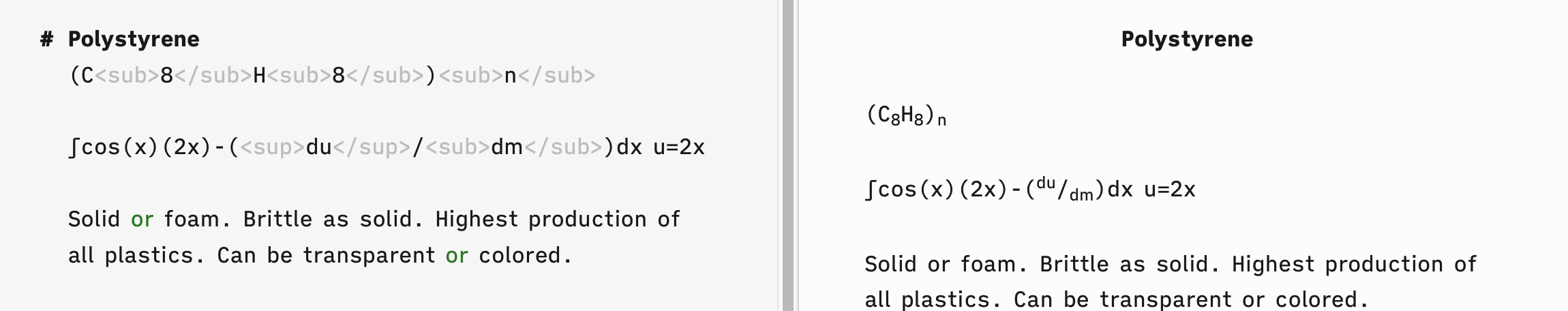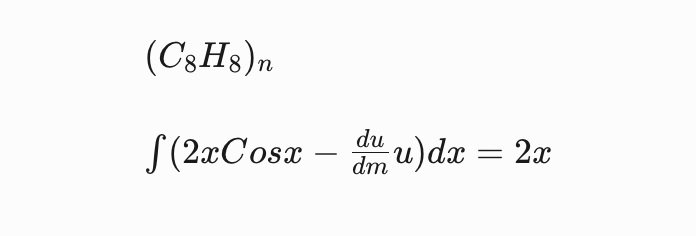# Is there a way to insert mathematical functions while writing a note in TA?

Hi everyone! This may be a dumb question, as I realize TA is supposed to work just with normal text files. But it seems if you were an engineer or a scientist, you might want to include mathematical equations in your notes. I'm thinking here about real equations, just as you might write them by hand, and ones that include commonly used Greek symbols, summation signs, integral signs, etc. I understand there is a way to include mathematical equations in some Markdown editors. Is this possible in TA?

• edited June 14

Yes, I have done this for propositional logic symbols. What I did was to google a list of the symbols, then created keyboard shortcuts in Keyboard Maestro to insert those symbols when I typed a specific trigger. e.g e§ gives ∃ for me.

edited to add: I wasn't using TA as my main note taking repository at the time, but I've just tried it out and it seems to work fine.

• @GBC I'm not sure if that will work for what I had in mind. A "normal" mathematical equation for me has integrals, differentials, items with both numerator and denominator, etc. It's not finding the symbols so much as how they are arranged in the equation. Does that make sense?

I would normally produce such an equation in MathCad or similar software, but then to reference that in a note, one would have to take a screenshot and insert it as an image - doable, but not ultimately that helpful.

There is a program called MathJax which produces equations that can be viewed in a web browser and I vaguely recall reading somewhere that there was a way of incorporating this into a Markdown document. OK; googling that topic yields the following information:

https://hiltmon.com/blog/2017/01/28/mathjax-in-markdown/

If someone has some experience with this approach, or another one, could they share it here?

• Does this help?
IA Writer - plain text on the left, preview mode on the right.Will Simpson
I'm a Zettelnant.
Research: Rationalism, Zen, Non-fiction Creative Writing
kestrelcreek.com

• @Will said:
Does this help?
IA Writer - plain text on the left, preview mode on the right.The proper syntax in iAWriter is actually simpler as in LaTeX;

$(C_8H_8)_n$

$\int(2xCosx-\frac{du}{dm}u)dx= 2x$

produces;• @GeoEng5 oh, of course, aplogies!

• Thank you both for that excellent information! I didn't understand that the capability to preview LaTeX was built into IA Writer.

After some searching around on my iPad, I realized I already had, a year or so ago, downloaded a very useful app called MathKey (it is billed as a LaTex converter). When you run MathKey, it acts as a whiteboard which will convert and send hand-written math equations to the following web site:

https://mathkey-app.com/

This is how it works: You go to the above web site and note the 6-digit number that lets you connect to a remote session. You start up MathKey on your iPad and enter the 6-digit number. Then you hand-write your mathematical equation on the iPad screen and hit "Send". On the web site, your equation shows up in beautiful mathematical symbols, with the associated LaTeX code and MathML code below.

The MathML code worked in IA Writer directly as copied from the MathKey web site but it is long, "bulky" and not that readable (at least to me). Initially the LaTeX code did not translate in IA Writer's preview mode, but I looked at your example above and saw I needed to add a $at the start and end of the LaTeX string. This is what MathKey gave me, before the addition of the$ signs:

\int \left( 2x\cos x-\frac {du} {dm}u\right) dx=2x

This LaTeX string is similar to but a bit "wordier" than the one shown by @Splattack. I don't really understand the details of LaTeX (hence why I'm using MathKey in the first place), but I can read through it enough to change a symbol if my handwriting isn't converted accurately.

Your responses have answered my question fully - thank you so much!! This will see a lot of application in my #Technical_knowledge notes.

• The Archive doesn't show MathJax preview (yet), but if you happen to have the app Marked installed, you can make use Marked to render MathJax very quickly.

Author at Zettelkasten.de • https://christiantietze.de/